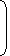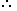# Tips and Tricks related to Stocks and Shares1. Stock Capital:The total amount of money needed to run the company is called the stock capital.
2. Shares or Stock:The whole capital is divided into small units, called shares or stock.For each investment, the company issues a ‘share-certificate’, showing the value of each share and the number of shares held by a person.The person who subscribes in shares or stock is called a share holder or stock holder.
3. Dividend:The annual profit distributed among share holders is called dividend.Dividend is paid annually as per share or as a percentage.
4. Face Value:The value of a share or stock printed on the share-certificate is called its Face Value or Nominal Value or Par Value.
5. Market Value:The stock of different companies are sold and bought in the open market through brokers at stock-exchanges. A share or stock is said to be:
1. At premium or Above par, if its market value is more than its face value.
2. At par, if its market value is the same as its face value.
3. At discount or Below par, if its market value is less than its face value.

Thus, if a Rs. 100 stock is quoted at premium of 16, then market value of the stock = Rs.(100 + 16) = Rs. 116.

Likewise, if a Rs. 100 stock is quoted at a discount of 7, then market value of the stock = Rs. (100 -7) = 93.

6. Brokerage:The broker’s charge is called brokerage.(i)  When stock is purchased, brokerage is added to the cost price.(ii) When stock is sold, brokerage is subtracted from the selling price.Remember:
1. The face value of a share always remains the same.
2. The market value of a share changes from time to time.
3. Dividend is always paid on the face value of a share.
4. Number of shares held by a person
 = Total Investment = Total Income = Total Face Value . Investment in 1 share Income from 1 share Face of 1 share
7. Thus, by a Rs. 100, 9% stock at 120, we mean that:
1. Face Value of stock = Rs. 100.
2. Market Value (M.V) of stock = Rs. 120.
3. Annual dividend on 1 share = 9% of face value = 9% of Rs. 100 = Rs. 9.
4. An investment of Rs. 120 gives an annual income of Rs. 9.
5. Rate of interest p.a   =   Annual income from an investment of Rs. 100
 =9 x 100% = 7 1 %. 120 2
In order to obtain an income of Rs. 650 from 10% stock at Rs. 96, one must make an investment of:
 A. Rs. 3100 B. Rs. 6240 C. Rs. 6500 D. Rs. 9600

Answer: Option B

Explanation:

To obtain Rs. 10, investment = Rs. 96.

 To obtain Rs. 650, investment = Rs.96 x 650= Rs. 6240. 10
A man buys Rs. 20 shares paying 9% dividend. The man wants to have an interest of 12% on his money. The market value of each share is:
 A. Rs. 12 B. Rs. 15 C. Rs. 18 D. Rs. 21

Answer: Option B

Explanation:

 Dividend on Rs. 20 = Rs.9 x 20= Rs. 9 . 100 5

Rs. 12 is an income on Rs. 100.Rs. 9 is an income on Rs.100 x 9= Rs. 15. 5 12 5

#### A 6% stock yields 8%. The market value of the stock is:

Correct! Wrong!

#### A man invested Rs. 4455 in Rs. 10 shares quoted at Rs. 8.25. If the rate of dividend be 12%, his annual income is:

Correct! Wrong!

#### Rs. 9800 are invested partly in 9% stock at 75 and 10% stock at 80 to have equal amount of incomes. The investment in 9% stock is:

Correct! Wrong!

#### By investing Rs. 1620 in 8% stock, Michael earns Rs. 135. The stock is then quoted at:

Correct! Wrong!

#### Sakshi invests a part of Rs. 12,000 in 12% stock at Rs. 120 and the remainder in 15% stock at Rs. 125. If his total dividend per annum is Rs. 1360, how much does he invest in 12% stock at Rs. 120?

Correct! Wrong!

QUIZ ON STOCKS & SHARES PROBLEMS IN APTITUDE
EXCELLENT!!!

Share your Results:

Don't miss out!
Subscribe To Our Newsletter

Learn new things. Get an article everyday.

Invalid email address
Give it a try. You can unsubscribe at any time.